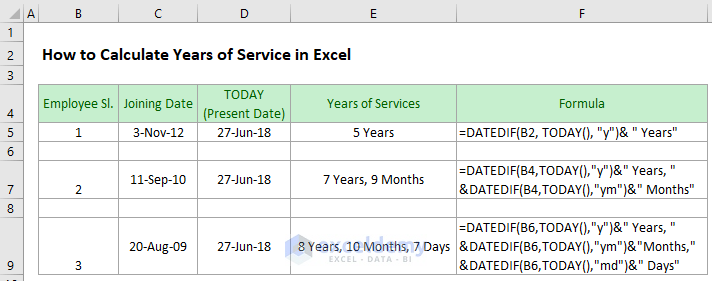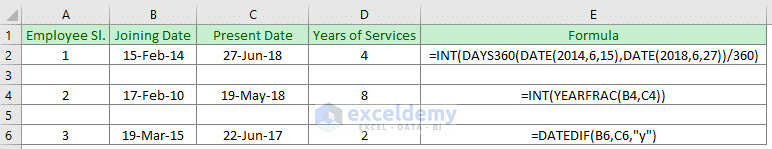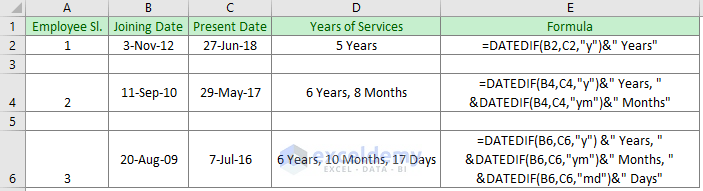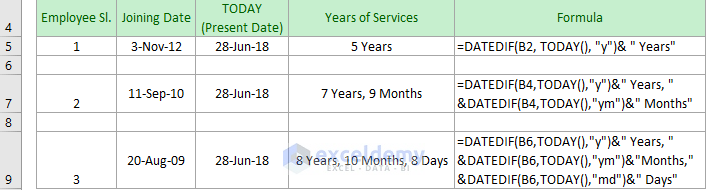Disclosure: This post may contain affiliate links, meaning when you click the links and make a purchase, we receive a commission.

# How to calculate years of service in Excel (3 easy ways)

Suppose you have an employee name who recently resigned from your company. You want to calculate his/her years of services at your company. There are many ways to calculate that. We can use many formulas for this. In this article, you will see the processes on how to calculate years of service in Excel. The length of service with days, months and years together will also be calculated.

Look into the below picture to see what we are going to perform.## Calculate Years of Service between Two Dates

As I said earlier there are many ways to calculate the years of services. Firstly, you will see this with some simple and short formulas. Look into the below picture where I calculated the years of services for different years by using different formulas.The formulas which are used here are given below.

• `=INT(DAYS360(DATE(2014,6,15),DATE(2018,6,27))/360)`
• `=INT(YEARFRAC(B4,C4))`
• `=DATEDIF(B6,C6,"y")`

In the first 2 formulas, the INT function has been used in the formulas which give the integer number avoiding the fractional number. In the last formula, we use an argument “y” for the DATEDIF function which gives the result as a full year between the two dates.

## Calculate the Length of Service in Excel between two specific dates

Now if you want to calculate the length of service of a person in years, months and days you can use the DATEDIF function. In this example, I calculated the years of service in three ways. The first one gives the output as years, the 2nd one gives the result as years and months and the 3rd one gives the full result with years, months, and days. Look into the below picture where all these results are carried out.Here the formulas which are used here are given below.

• `=DATEDIF(B2, C2, "y")& " Years"`
• `=DATEDIF(B4,C4,"y")&" Years, "&DATEDIF(B4,C4,"ym")&" Months"`
• `=DATEDIF(B6,C6,"y") & " Years, " & DATEDIF(B6,C6,"ym") & " Months, " & DATEDIF(B6,C6,"md") & " Days"`

## Calculate the Length of Service between a Previous Date and Present Date

There is a built-in function in Excel that can give you the present date. This function is the TODAY function. It is written in Excel as, =TODAY (). This function is categorized as Date/Time function in Excel. It can be used in a formula also. Like, in the previous examples we worked with some random dates. Instead of these random dates if you want to find out the length/years of service for a previous date and present date you have to insert the TODAY function instead of the later dates which are placed in column C. Let`s look into the below picture to get a whole idea.Instead of using the TODAY function in the formula, you can use this in a cell, and in your formula, you can refer it. The formulas which are used here are given below.

• `=DATEDIF(B2, TODAY(), "y")& " Years"`
• `=DATEDIF(B4,TODAY(),"y")&" Years, "&DATEDIF(B4,TODAY(),"ym")&" Months"`
• `=DATEDIF(B6,TODAY(),"y") & " Years, " & DATEDIF(B6,TODAY(),"ym") & " Months, " & DATEDIF(B6,TODAY(),"md") & " Days"`
Note: The advantage of using the TODAY function is that it gets updated every time you open your worksheet. So, every day you open your worksheet the length of service also gets updated.

## Conclusion

In this article, basically, we calculate the number of years between two dates in Excel. The DATEDIF function makes it easier to calculate the length between two dates. Hope you did not face any difficulties while reading this article. Be connected with us to get more articles.

## Related Articles

Hello!

Welcome to my Profile. Here I will be posting articles related to Microsoft Excel. I have completed my BSc in Electrical and Electronic Engineering from American International University-Bangladesh. I am a diligent, goal-oriented engineer with an immense thirst for knowledge and attitude to grow continuously. Continuous improvement and life-long learning is my motto.

1. ReplyChamila Oct 1, 2019 at 9:16 AM

Very useful formula for HR Professionals.

• ReplyOct 1, 2019 at 10:30 AM

2. ReplyAraflor Jun 23, 2020 at 8:27 AM

This was very helpful! Great information! Thank you very much!

3. ReplyLuluBelle Sep 17, 2020 at 1:29 AM

Thanks so much! This made things a whole lot easier for me. As Chamila said, very useful formula for HR folks.

• ReplySep 17, 2020 at 10:56 AM